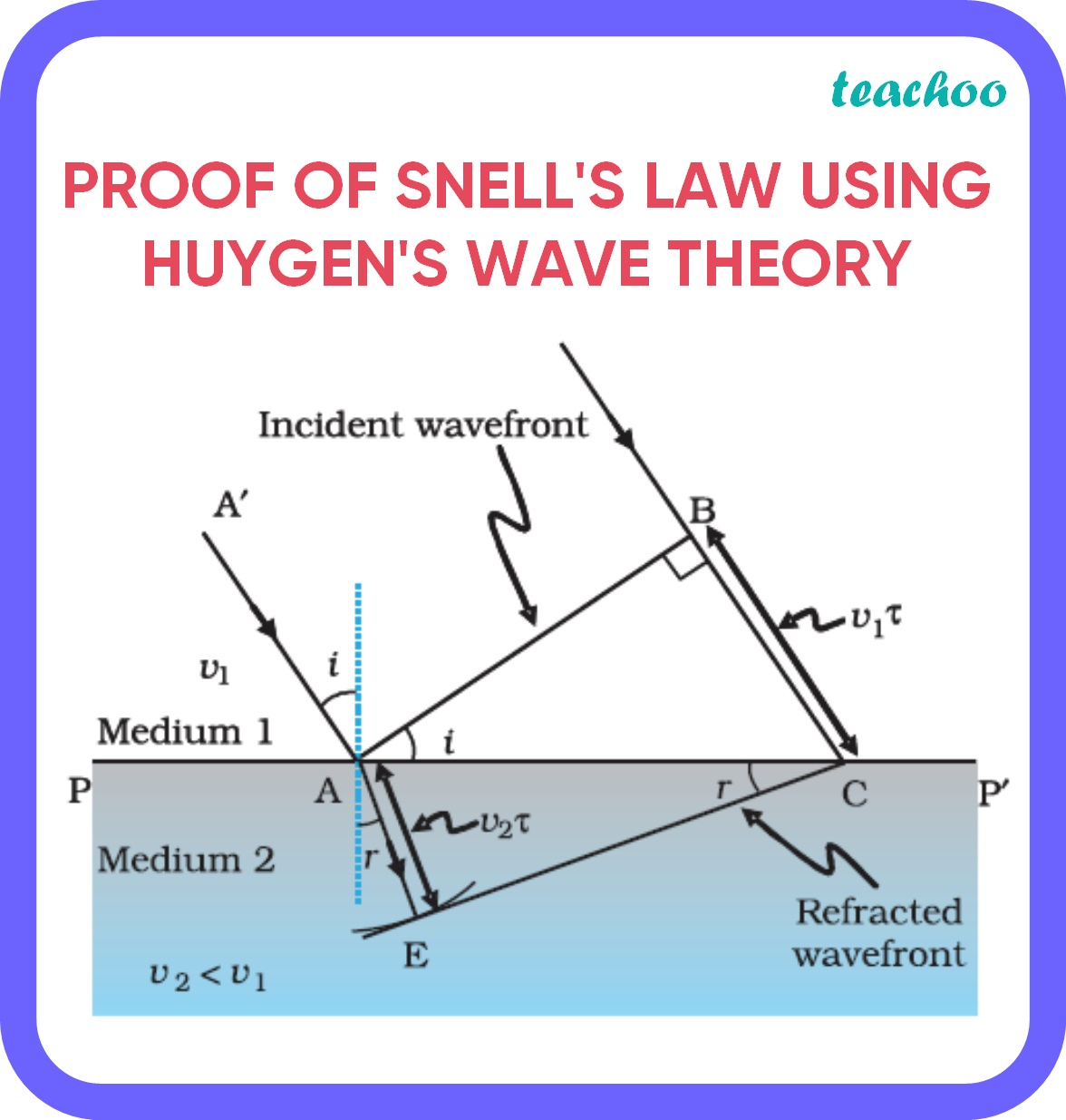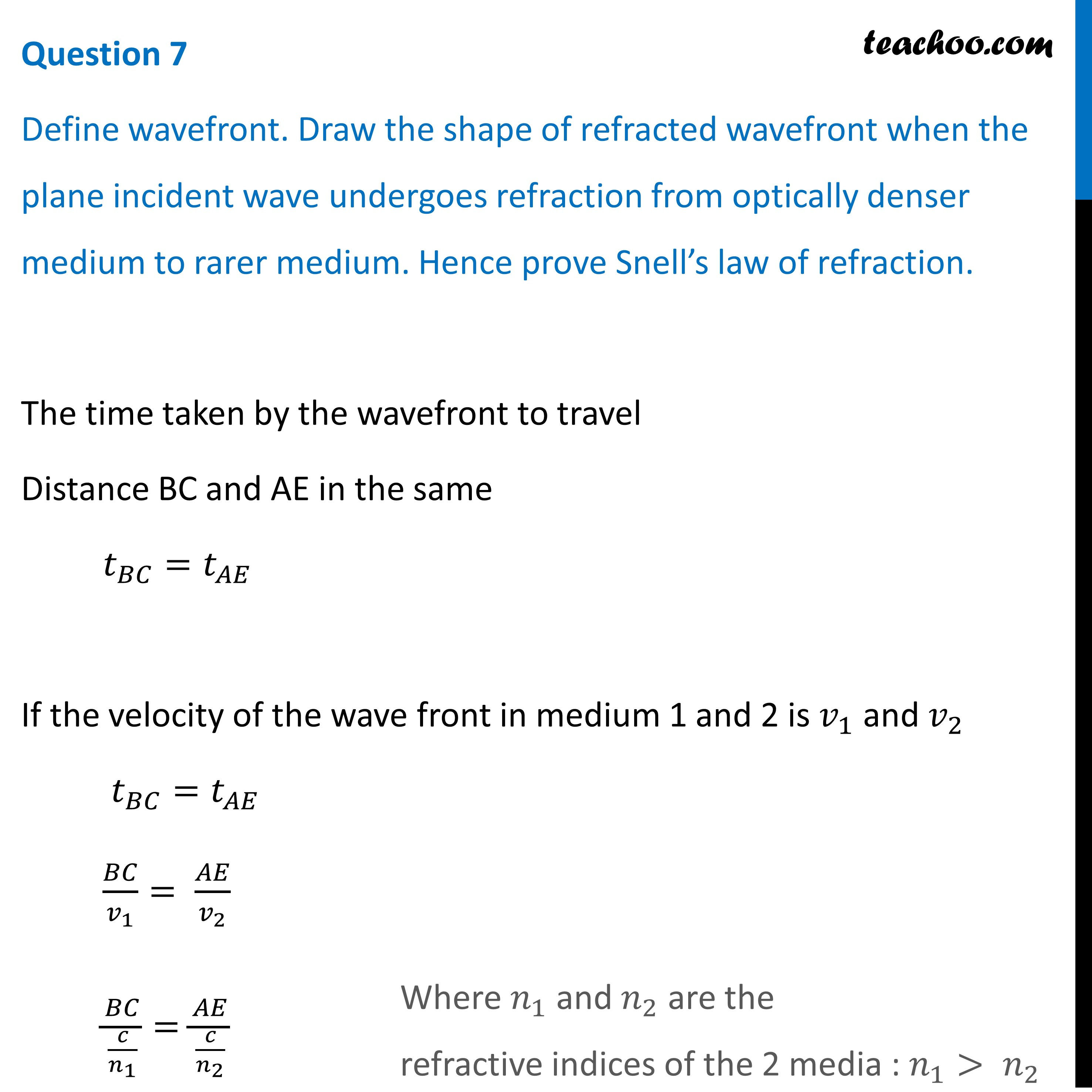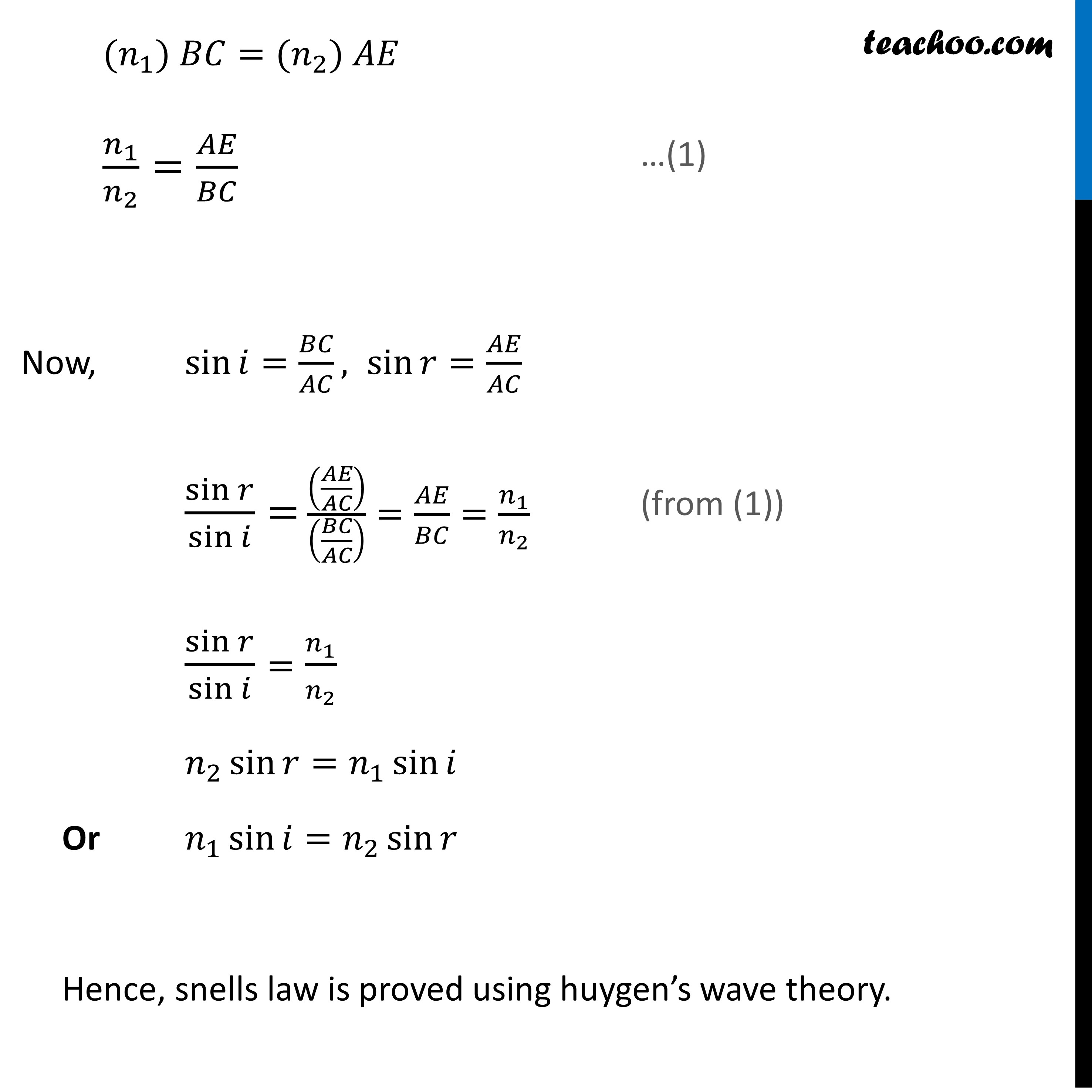CBSE Class 12 Sample Paper for 2022 Boards [Term 2] - Physics

Physics Class 12
Solutions to CBSE Sample Paper - Physics Class 12

## Define wavefront. Draw the shape of refracted wavefront when the plane incident wave undergoes refraction from optically denser medium to rarer medium. Hence prove Snell’s law of refraction.

A locus of points in space which oscillate in the same phase is called a wavefront

That is, the phase difference between any two points of the same wavefront is zero .

Let us assume an incident wavefront AB incident on an interface between 2 media with refraction indices n1>n2.According to Huygen’s wave theory:

• A source of light  gives out waves in all directions.
• Every point on the wavefront is a source of disturbance. These disturbances called secondary wavelets,  travel in all directions.
• The time taken by secondary wavelets to travel from primary to secondary wavefront is a constant

Therefore, on the basis on these statements we can say that:Learn in your speed, with individual attention - Teachoo Maths 1-on-1 Class

### Transcript

Question 7 Define wavefront. Draw the shape of refracted wavefront when the plane incident wave undergoes refraction from optically denser medium to rarer medium. Hence prove Snell’s law of refraction. The time taken by the wavefront to travel Distance BC and AE in the same 𝑡_𝐵𝐶=𝑡_𝐴𝐸 If the velocity of the wave front in medium 1 and 2 is 𝑣_1 and 𝑣_2 𝑡_𝐵𝐶=𝑡_𝐴𝐸 𝐵𝐶/𝑣_1 = 𝐴𝐸/𝑣_2 𝐵𝐶/(𝑐/𝑛_1 )=𝐴𝐸/(𝑐/𝑛_2 ) 〖(𝑛〗_1) 𝐵𝐶=〖(𝑛〗_2) 𝐴𝐸 𝑛_1/𝑛_2 =𝐴𝐸/𝐵𝐶 Now, sin⁡𝑖=𝐵𝐶/𝐴𝐶, sin⁡𝑟=𝐴𝐸/𝐴𝐶 sin⁡𝑟/sin⁡𝑖 = ((𝐴𝐸/𝐴𝐶))/((𝐵𝐶/𝐴𝐶) )=𝐴𝐸/𝐵𝐶=𝑛_1/𝑛_2 sin⁡𝑟/sin⁡𝑖 =𝑛_1/𝑛_2 𝑛_2 sin⁡𝑟=𝑛_1 sin⁡𝑖 Or 𝑛_1 sin⁡𝑖=𝑛_2 sin⁡𝑟 Hence, snells law is proved using huygen’s wave theory.